## Plus Blog

July 29, 2015

Are you queueing in an airport, wondering if there is a better way to deal with the mass of humanity trying to board planes? Are you stuck at home wondering where the summer weather has gone? Would you like to see our information overloaded world in a more beautiful way? Do you think the world would be a better place if we made lovely maths, instead of war? Then you should book your place for the 2015 British Science FestivalMathematical love!

This year the festival is in Bradford from 7-10 September. And, as well as delights in all areas of science, there is some fascinating maths to enjoy. In Advanced airports and on-time ambulances, Kevin Glazebrook and Vincent Knight will explain how number crunching improve holiday travel chaos in airports, and get you medical help in an emergency quicker. And on the subject of health, you can join in a mini-epidemic with Erin Lafferty as she reveals the medical secrets behind infection in Can maths decode infectious diseases?

Meanwhile scientist Martin Brinkworth, mathematician Simon Shepherd and artist Marcus Levine will show us the beautiful side of big data in Art and big data and explore what our innate appreciation of aesthetics in these representations can reveal. In Love and war: the mathematical way Hannah Fry will demonstrate the power of maths in understanding human behaviour in the rawest of human emotions. You can find out more about these and all the other fascinating events at the Festival website – booking is now open!

To get you in the mood, you can read more about the maths behind infectious diseases, art, love and war on Plus.

May 26, 2015

On May 23rd John Nash sadly died in a car crash, along with his wife Alicia. To the wider world Nash was famous as the main character of the book and film A beautiful mind, and to mathematicians for his important work in game theory, geometry and the theory of differential equations. Nash won the Nobel Prize for Economics in 1994 and was awarded the prestigious Abel Prize only this year. Here are three Plus articles that shed light on some of his work.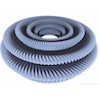The Abel Prize 2015: All wrapped up — This article explores Nash's work in geometry and differential equations, which won him a share of the Abel Prize 2015.If we all go for the blonde — A gentle introduction to some game theory, based on a scene from the film A beautiful mind.Game theory and the Cuban missile crisis — A fascinating application of game theory, including the concept of a Nash equilibrium, to a dangerous political stand-off.

To find out more about the life and work of John Nash read his biography on the MacTutor History of Mathematics archive.

April 21, 2015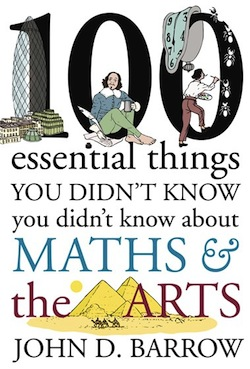Barrow's new book.

Ever wondered why diamonds sparkle? Or why an egg is egg shaped?

John D. Barrow, mathematician, cosmologist and boss of Plus, explores the answers in a public talk at the Bradford Literature Festival on Sunday May 17. Taking us on a 100-step tour through art forms as various as sculpture, literature, architecture and dance, he reveals what maths can tell us about the mysteries of the worlds of art and design. (To get a taste, read Barrow's Plus article Where to stand to look at sculptures)

The talk is from 11am to 12pm at the City Library in Bradford and admission is £6. You can book tickets here.

April 1, 2015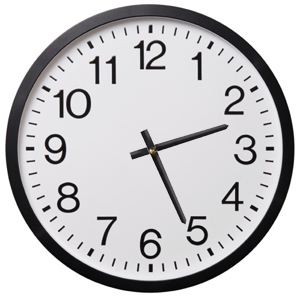You do modular arithmetic several times every day when you are thinking about time. Imagine, for example, you're going on a train trip at 11pm that lasts three hours. What time will you arrive? Not at 11+3 = 14 o'clock, but at 2 o'clock in the morning. That's because, on a 12-hour clock, you start counting from the beginning again after you get to 12. (On a 24-hour clock, you start again after you get to 24.) So, on a 12-hour clock you have:

4 + 9 = 1,
7 + 7 = 2,
5 + 12 = 5,

and so on. When you are subtracting hours, you do the same but backwards:

4 - 7 = 9
1 - 11 = 2
6 - 12 = 6.

You could play the same game using other numbers, apart from 12 and 24, to define your cycle. For example, in modular arithmetic modulo 5 you have

4 + 2 = 1
3 + 4 = 2
1 - 4 = 2
3 - 5 = 3

These sums can be a little tedious to work out if you're counting on your fingers, but luckily there is a general method. Let's say you're doing arithmetic modulo some natural number p and you're looking at some other natural number x. To find the value of x modulo p (the value of x on a clock with p hours), compute the remainder when dividing x by p: that's your result.

This also works when x is negative (noting that the remainder is defined to be always positive). For example, for p = 12 and x = -3 we have

-3 = (-1) x 12 + 9,

so the remainder is 9. Therefore -3 modulo 12 is equal to 9. (If you use the modulus function in some computer languages you have to be a little careful though, as some return a different value for negative numbers.)

If you want to add or subtract two numbers module some natural number p, you simply work out the result, call it x, in ordinary arithmetic and then find the value of x modulo p.

There is clearly something very cyclical about modular arithmetic. Whatever number p defines your arithmetic, you can think of it as counting forward or backward in clock with p hours. To put this in technical maths language, modular arithmetic modulo p gives you a cyclic group of order p. You can find out more about group theory in this article and about modular arithmetic on our sister site NRICH.

March 27, 2015One way to start getting your head around groups, those slightly scary abstract structures that belong to the field of algebra, is to think of a 12-hour clock. You can add hours on this clock, for example

2 o'clock + 4 hours = 6 o'clock
8 o'clock + 5 hours = 1 o'clock
3 o'clock + 12 hours = 3 o'clock

and so on. One thing to notice here is that however many hours you add, the answer is always going to be a number between 1 and 12. In this sense, addition on a 12-hour clock is closed: it never gets you out of the 1 to 12 land.

Another thing to notice is that adding 12 hours gets you back to where you started (it's the same as doing nothing): for any starting time a, we have

a + 12 = a.

This is why you could equally well write 0 for the number 12 in our clock-world.

A third interesting feature is that when you have added some number a of hours, you can get back to where you started by adding another 12 - a hours. For example, starting at 1 o'clock and adding 7 gives

1 + 7 = 8.

and then adding another 12 - 7 = 5 hours gives

8 + 5 = 1.

So adding a and then 12 - a is the same as doing nothing (or, equivalently, adding 12).

Taking a small step into abstraction, we can describe our 12-hour arithmetic as follows. We have a set S (which consists of the numbers 1 to 12) and a binary operation that combines any two elements of S to give a third (the operation is addition modulo 12 in our example). The set and the operation satisfy the following three rules:

• Whenever you add two elements of the set S the result is also an element of S (addition is closed).
• There is an element of S (in our case the number 12), such that when you add that element to any other element a of S, the result is a. This element is called the identity of S.
• For every element a of S there is another element b of S, so that a + b is equal to the identity of S. The element b is called the inverse of a. (In the clock example the inverse of a is 12 - a.)

There is also a fourth rule satisfied by our set and operation:

• For three elements a, b and c of S we have (a + b) + c = a + (b + c). In other words, it doesn't matter whether you add the third number to the sum of the first two, or whether add the sum of the last two numbers to the first number.

Move the slider to rotate the 12-gon.

There are many other structures that also satisfy these rules. As an example, think of a regular 12-gon, as the one pictured on the left. You can rotate this shape clockwise around its centre by 30 degrees and what you end up with is the same shape as the one you started with. The rotation is a symmetry of the 12-gon. Now let our set S consist of all the clockwise rotations through multiples of 30 degrees. So that's 30 degrees, 60 degrees, 90 degrees, etc, all the way up to 330 degrees and, finally, the full turn. (All of these are symmetries of the 12-gon.) Think of adding two rotations as doing one after the other: so the rotation through 60 degrees plus the rotation through 90 degrees equals the rotation through 150 degrees.

This structure forms a group. Addition is closed because when you follow one clockwise rotation through a multiple of 30 degrees by another, the result is also a clockwise rotation through a multiple of 30 degrees. There's also an identity element, namely the rotation through 360 degrees. For every rotation there is an inverse rotation, so that combining the two is the same as doing the identity rotation. For example, the inverse of the rotation through 30 degrees is the rotation through 330 degrees.

In general, a group is a set S of elements together with a binary operation that satisfies the four rules above. Our two examples here are finite groups in that the set S has finitely many elements. But there are also infinite groups. You can try to convince yourself that the set of whole numbers (including the negative ones) with the operation of addition forms an infinite group.

It's interesting to note that two groups can consist of different objects and involve different operations but still have the same structure. By an unbelievably lucky coincidence, the two groups we have seen so far are an example of this. In our clock face example above, adding one hour corresponds to turning the clock hand clockwise through a twelfth of a turn — that's 30 degrees. Adding b hours corresponds to turning the hand through b twelfths of a full turn — that's b x 30 degrees. In fact, the elements of the clock face group (the numbers 1 to 12) behave under their modular addition in exactly the same way as the elements of our 12-gon rotation group do under their own notion of addition. If, in our clock face group, a + b = c, then you know immediately that in the rotation group the rotation through a x 30 degrees followed by the rotation through b x 30 degrees gives the rotation through c x 30 degrees. And vice versa. The two groups are said to be isomorphic. (See here for a technical definition.) Mathematicians often find that a group that arises in one context is isomorphic to a group they know from a very different context.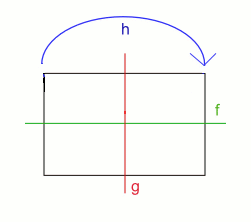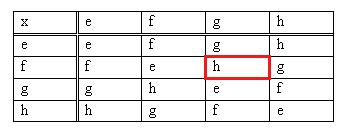This table represents a group called the Klein 4-group.

This is why it can make sense to think of a group, not as made up of rotations or numbers or some other explicit type of objects, but as a collection of abstract objects (we can use letters to denote these objects) that combine in a particular way under the binary operation. You can keep track of how they combine in a table, such as the one on the left. To see the result of the sum f + g, find the cell corresponding to row f and column g. Its entry is h, so f + g = h. The symbol e in this table stands for the group identity.

The group described by this table is known as the Klein 4-group. It's isomorphic to the symmetry group of a rectangle, writing e for the identity symmetry (doing nothing), f for the reflection in the horizontal axis, g for the reflection in the vertical axis and h for the rotation through 180 degrees. Using this abstract representation, you can describe groups that are isomorphic, even if they arise in very different contexts, in one go. That's the power of abstraction!